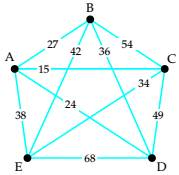# Use the greedy algorithm to find a Hamiltonian circuit starting at vertex A in the weighted### Mathematical Excursions (MindTap C...

4th Edition
Richard N. Aufmann + 3 others
Publisher: Cengage Learning
ISBN: 9781305965584

#### Solutions

Chapter
Section### Mathematical Excursions (MindTap C...

4th Edition
Richard N. Aufmann + 3 others
Publisher: Cengage Learning
ISBN: 9781305965584
Chapter 5.2, Problem 13ES
Textbook Problem
281 views

## Use the greedy algorithm to find a Hamiltonian circuit starting at vertex A in the weightedTo determine

To determine a Hamiltonian circuit starting at vertex A in the weighted graph using the greedy algorithm.

### Explanation of Solution

Given information:

Given, weighted graph is:

Calculation:

A path in a Hamiltonian graph is said to be a Hamiltonian Circuit if it begins and ends at the same vertex and passes through each vertex of a graph exactly once.

The greedy algorithm states to travel along a connected edge that has the smallest weight that is not yet visited. This process continues till all the vertices are visited and returned to the starting vertex.

Here, the vertex A is selected.

Vertex A is of degree 4 and the weights of the connected edges are 27, 15, 24, 38

Hence, as per the Greedy Algorithm the edge with weight 15 is selected i.e. path reaches C.

Vertex C is of degree 4 and the weights of the connected edges are 54, 15, 34, 49

The least of the weights is 15 but it leads to vertex A which is already visited.

Hence, as per the Greedy Algorithm the edge with weight 34 is selected i...

### Still sussing out bartleby?

Check out a sample textbook solution.

See a sample solution

#### The Solution to Your Study Problems

Bartleby provides explanations to thousands of textbook problems written by our experts, many with advanced degrees!

Get Started

Find more solutions based on key concepts
Solve the equations in Exercises 112 for x (mentally, if possible). x+1=2x+2

Finite Mathematics and Applied Calculus (MindTap Course List)

Let f be the function defined by f(x) = 5x + 6. Find f(3), f(3), f(a), f(a), and f(a + 3).

Applied Calculus for the Managerial, Life, and Social Sciences: A Brief Approach

The area of the shaded region is given by:

Study Guide for Stewart's Multivariable Calculus, 8th

Solve for x: e2x1 = 10. a) 12(1+10e) b) 3 + ln 10 c) 2 + ln 10 d) 1+ln102

Study Guide for Stewart's Single Variable Calculus: Early Transcendentals, 8th